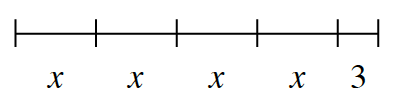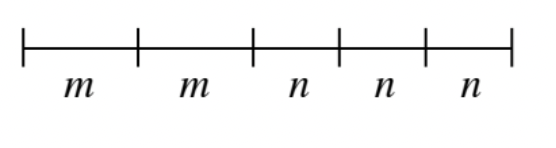### Home > CCAA > Chapter 3 Unit 2 > Lesson CC2: 3.2.1 > Problem3-37

3-37.

Jorge was thinking about using variables to represent lengths of a tightrope walker’s many tricks.

1. Jorge wrote the expression $x+x+x+x+3$ to represent the sequence shown in the diagram at right. Does his expression make sense? Explain.

Try thinking about how you might write this expression first. Is it different from Jorge's?

Yes, this expression does make sense. Why do you think so?
Jorge explained why he chose this expression and said, ''I chose this expression because the tightrope is divided into separate parts, and I wanted to write one expression that could combine all parts into one. The $x$'s and the $3$ are all different lengths that make the tightrope a whole. So, by adding the lengths together, the total length could be found.''1. Write an expression to represent the sequence shown in the diagram at right.

Together, all of these letters represent the entire length of the rope. How can you combine them into one expression?1. In part (a), if $x=5$ feet, how long is the tightrope?

Use Jorge's expression and substitute $5$ in for the $x$ 's. Can you now find the value of the expression?

2. In part (b), if $m = 3$ feet and $n = 2$ feet, how long is the tightrope?

Using the expression you wrote in part (b), try substituting the $m$'s with $3$'s and $n$'s with $2$'s.

Your expression should have looked something like this: $m+m+n+n+n$ or $2m+3n$. Can you find the length now?

The tightrope is $12$ feet long.